# Solution and Gradient Plots with `pdeplot` and `pdeplot3D`

### 2-D Solution and Gradient Plots

To visualize a 2-D scalar PDE solution, you can use the `pdeplot` function. This function lets you plot the solution without explicitly interpolating the solution. For example, solve the scalar elliptic problem $-\nabla u=1$ on the L-shaped membrane with zero Dirichlet boundary conditions and plot the solution.

Create the PDE model, 2-D geometry, and mesh. Specify boundary conditions and coefficients. Solve the PDE problem.

```model = createpde; geometryFromEdges(model,@lshapeg); applyBoundaryCondition(model,"dirichlet", ... "Edge",1:model.Geometry.NumEdges, ... "u",0); c = 1; a = 0; f = 1; specifyCoefficients(model,"m",0,"d",0,"c",c,"a",a,"f",f); generateMesh(model); results = solvepde(model);```

Use `pdeplot` to plot the solution.

```u = results.NodalSolution; pdeplot(model,"XYData",u,"ZData",u,"Mesh","on") xlabel("x") ylabel("y")```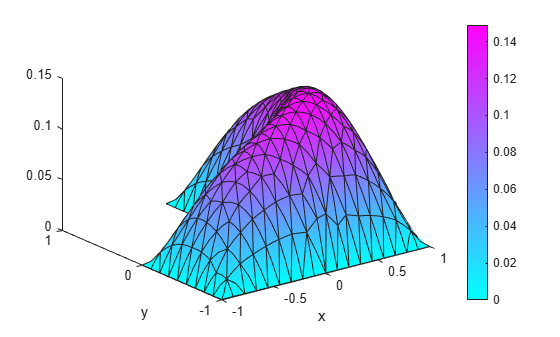To get a smoother solution surface, specify the maximum size of the mesh triangles by using the `Hmax` argument. Then solve the PDE problem using this new mesh, and plot the solution again.

```generateMesh(model,"Hmax",0.05); results = solvepde(model); u = results.NodalSolution; pdeplot(model,"XYData",u,"ZData",u,"Mesh","on") xlabel("x") ylabel("y")```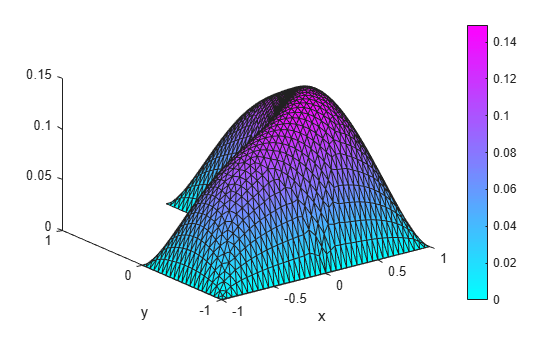Access the gradient of the solution at the nodal locations.

```ux = results.XGradients; uy = results.YGradients;```

Plot the gradient as a quiver plot.

`pdeplot(model,"FlowData",[ux,uy])`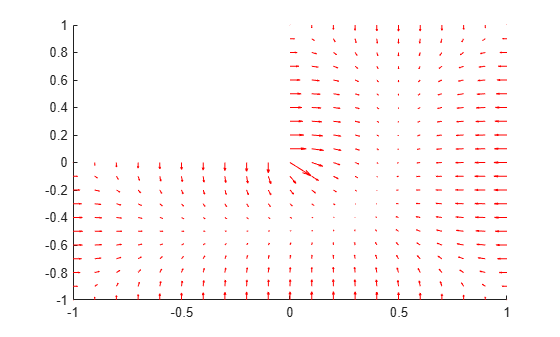### 3-D Surface and Gradient Plots

Obtain a surface plot of a solution with 3-D geometry and N > 1.

First, import a tetrahedral geometry to a model with N = 2 equations and view its faces.

```model = createpde(2); importGeometry(model,"Tetrahedron.stl"); pdegplot(model,"FaceLabels","on","FaceAlpha",0.5) view(-40,24)```Create a problem with zero Dirichlet boundary conditions on face 4.

`applyBoundaryCondition(model,"dirichlet","Face",4,"u",[0,0]);`

Create coefficients for the problem, where `f = [1;10]` and `c` is a symmetric matrix in 6N form.

```f = [1;10]; a = 0; c = [2;0;4;1;3;8;1;0;2;1;2;4]; specifyCoefficients(model,"m",0,"d",0,"c",c,"a",a,"f",f);```

Create a mesh for the solution.

`generateMesh(model,"Hmax",20);`

Solve the problem.

```results = solvepde(model); u = results.NodalSolution;```

Plot the two components of the solution.

```pdeplot3D(model,"ColorMapData",u(:,1)) view(-175,4) title("u(1)")```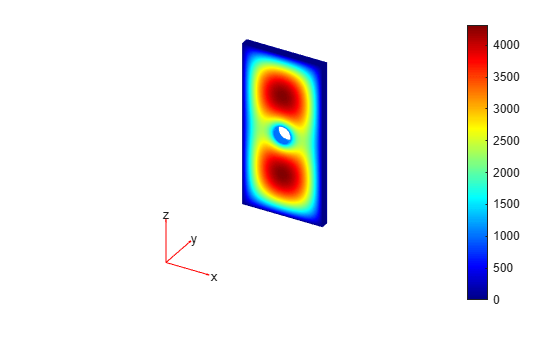```figure pdeplot3D(model,"ColorMapData",u(:,2)) view(-175,4) title("u(2)")```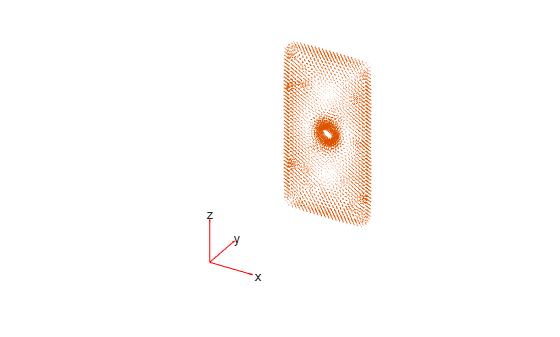Compute the flux of the solution and plot the results for both components.

```[cgradx,cgrady,cgradz] = evaluateCGradient(results); figure pdeplot3D(model,"FlowData",[cgradx(:,1) cgrady(:,1) cgradz(:,1)])``````figure pdeplot3D(model,"FlowData",[cgradx(:,2) cgrady(:,2) cgradz(:,2)])```